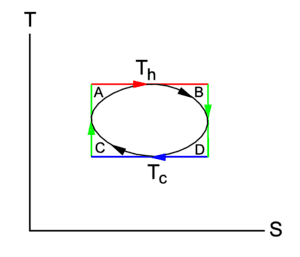# Thermodynamic cycle efficiency

• TK421

## Homework Statement

n moles of gas, that follow van der Waals equation are to be employed as the auxiliary system in a circular cycle(parameterized using T_h and T_c as shown on the TS diagram. Calculate the efficiency of the cycle.## Homework Equations,, Q = TdS

## The Attempt at a Solution

Idea is simple. I just divide circle curve horizontally in two two curves. Using Q = TdS , by integration over the top curve i obtain Q_(in), by integrating over the bottom curve i obtain Q_(out). Then i easily get efficiency using equation above. The only problem is, that i don't know, how to calculate those integrals with only van der Waals equation of state initially given.

#### Attachments

What is the equation for the area of an ellipse in terms of the semi major and semi minor axes?

Sorry, I wasn't precise enough, it has to be a circle, not an ellipse. I put that diagram more for an illustrative purpose.

Sorry, I wasn't precise enough, it has to be a circle, not an ellipse. I put that diagram more for an illustrative purpose.
How can it be a circle if the units are different? If you change the units of either T or S, the shape changes.

Absolutely.
The task itself states, that we are given a cycle, which is represented as a circle on a T-S diagram, which is parameterized using T_h and T_c.
My main concern though is, how would i get an expression of T as a function of S...

Absolutely.
The task itself states, that we are given a cycle, which is represented as a circle on a T-S diagram, which is parameterized using T_h and T_c.
My main concern though is, how would i get an expression of T as a function of S...
$$\left(\frac{T-\frac{(T_H+T_C)}{2}}{\frac{(T_H-T_C)}{2}}\right)^2+\left(\frac{S-\frac{(S_{max}+S_{min})}{2}}{\frac{(S_{max}-S_{min})}{2}}\right)^2=1$$

Like I said, since T and S have different units, you can't represent the variation as a circle on a T-S diagram. What don't you understand about this?

I do understand it now. Thank you :)

I do understand it now. Thank you :)
So what do you get for the efficiency?